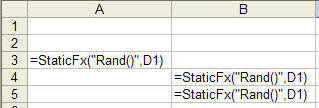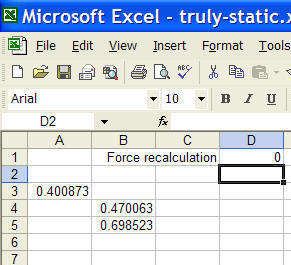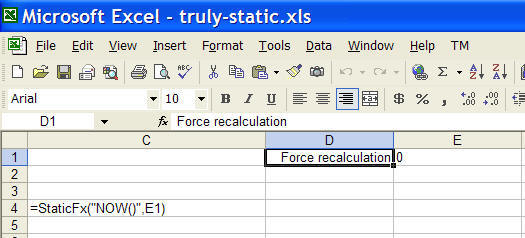You are on the Home/Excel/VBA/Static Random Values pageShare Your

# Static function values

There are instances when one needs a function that yields a result that is not controlled by Excel's recalculation engine.  For example, there are instances when a date, once added to a document, should remain unchanged.  Similarly, there are instances when one wants a random number to change only when required for business / application purposes, and not every time Excel recalculates formulas.  Some of the simpler techniques that one finds will often fail when Excel is forced to recalculate everything -- on a Windows platform with CTRL+SHIFT+ALT+F9.

There are two ways to accomplish this goal.  The first requires enabling iterative calculations -- and that comes with its own set of quirks.  The other is to shift the responsibility of recalculation from Excel into user written code!

### Using Iterative Calculations

To turn on iterative calculations, select Tools | Options... | Calculation tab.  Check on the box for Iteration.

Now, suppose cell E1 controls whether or not a new value is to be calculated.  If it contains FALSE, no change should be made to the existing value.  If it is TRUE, a new function value is desired.

To generate a random number using this technique, in some cell, say C3, enter the formula =IF(E1,RAND(),C3)  Set E1 to TRUE and C3 will contain a new random number each time Excel recalculates the worksheet.  Set E3 to FALSE and the last random number will remain unchanged.  Not even a force full recalculation (CTRL+SHIFT+ALT+F9) will change that value!

To use this technique with today's date, use DATE() instead of RAND() in the formula in C3.

### Assuming responsibility for recalculations -- writing static functions

The idea behind this code is to remember the old values of cells until the user requests that they be replaced by a newer value.  This is done by saving information about which cells are affected by this technique as well as the current value of those cells.  The information is saved on a worksheet by worksheet basis using worksheet level names.  While the original code started off with the idea of providing only a static random function, it quickly evolved into a technique that can be applied to any Excel function.

Note that this technique currently works only with formulas that return a numeric value.

The code below has not been extensively tested and its reliability is unknown when formulas in one worksheet use values from static functions in another worksheet.  In such instances, you use the method at your own risk.

### How is this technique used?

Suppose in one sheet, three random numbers are needed such that they change only when the user wants them to.  Similarly, in another worksheet, a single cell should contain the current date and time.  However, this should change only when the user indicates that a change is needed.

In the first case, where the RAND() function is to be used in a static fashion, suppose cell D1 will control when the formulas should be recalculated.  Three random numbers are needed -- in A3, B4, B5.  Enter the formulas as shown as Figure 1.  Change D1 to TRUE (or 1) and the values will change each time Excel recalculates the worksheet.  Change D1 to 0 and the values will remain unchanged.Note that the actual function, RAND() is passed as a string argument to StaticFx.  The control cell, D1, is the 2nd argument.

Similarly, in the other worksheet where the the cell contains the date and time, suppose C4 contains the static time and E1 controls when the value should change.  The formulas are shown in Figure 2.  Again, the actual function, DATE() is passed as a string argument to the StaticFx function and the control cell is the 2nd argument.### Making it work

In the Workbook code module, enter

 `Option Explicit` ```Private Sub Workbook_Open() ReDim AlreadyDone(0 To 0) ReDim OldValues(0 To 0) End Sub```

In a standard code module (optionally, named PublicFX), enter

 ```Option Explicit Function StaticRand(Optional ForceRecalc As Boolean = False) As Variant Dim MatchRslt If Application.Caller.Cells.Count > 1 _ Or Application.Caller.Areas.Count > 1 Then StaticRand = "This function must be entered in only one cell at a time" Exit Function '<<<<< End If On Error Resume Next MatchRslt = Application.WorksheetFunction.Match( _ Application.Caller.Address, AlreadyDone, 0) On Error GoTo 0 If IsEmpty(MatchRslt) Then MatchRslt = UBound(AlreadyDone) AlreadyDone(MatchRslt) = Application.Caller.Address OldValues(MatchRslt) = Rnd() MatchRslt = MatchRslt + 1 ReDim Preserve AlreadyDone(MatchRslt) ReDim Preserve OldValues(MatchRslt) ElseIf ForceRecalc Then OldValues(MatchRslt - 1) = Rnd() End If StaticRand = OldValues(MatchRslt - 1) End Function```

In a standard code module (optionally, named SupportModule), enter

 ```Option Explicit Option Private Module Public AlreadyDone() As String Public OldValues() As Double```